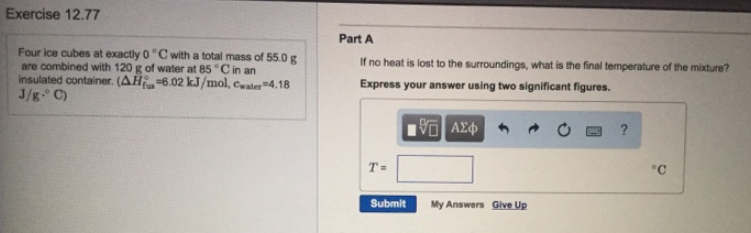# Problem: Four ice cubes at exactly 0°C with a total mass of 55.0 g are combined with 120 g of water at 85°C in an insulated container. (ΔHfun° = 6.02 kJ/mol, cwater = 4.18 J/g • °C) Part AIf no heat is lost to the surroundings, what is the final temperature of the mixture? Express your answer using two significant figures.

###### FREE Expert Solution
80% (435 ratings)###### Problem Details

Four ice cubes at exactly 0°C with a total mass of 55.0 g are combined with 120 g of water at 85°C in an insulated container. (ΔHfun° = 6.02 kJ/mol, cwater = 4.18 J/g • °C)

Part A

If no heat is lost to the surroundings, what is the final temperature of the mixture?